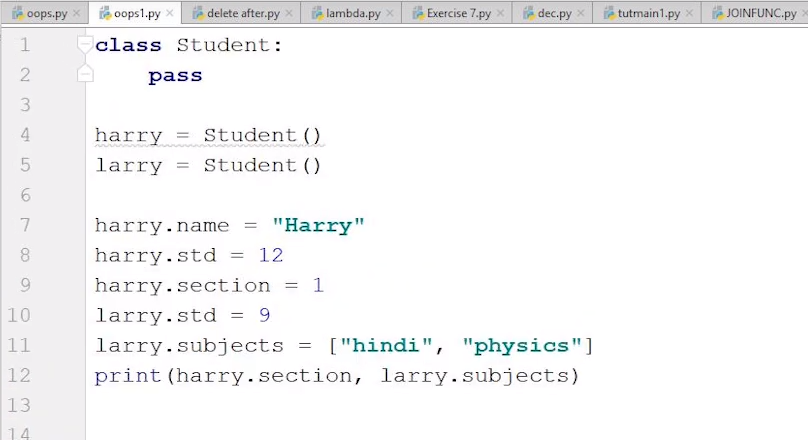### Instance & Class Variables | Python Tutorials For Absolute Beginners In Hindi #54

In the previous tutorial, we learned about the object and their working with variables in detail. Here we have created a class Student. We also created its two objects (harry and larry) and also a few of their instance variables as well.When working with objects in Python, there are two types of variables we have to work with i.e. instance variables and class variables. But what do these types of variables mean, and how do they work? OOP allows the variables to be used at the class level or the instance level. In this tutorial, we are going to learn about the two different kinds of variables associated with a class and the difference between them. The variables are:

• Instance variable
• Class variable

#### Instance variable:

"Instance variables are the variables for which the value of the variable is different for every instance."

We can also say that the value is different for every object that we create. Let us dive into some in-depth explanation. When we create a class, we define a few variables along with it. For example, we have created a class of Students, and we have defined a variable age. All the students cannot have the same age in a class, so we have assigned the variable an average age of 16. Now, whenever we use an object to print the value of age, it will show 16. We can try to change the value of age, but it will create a new instance variable for the specific object that we are updating it for, hence defining the value to it.

The code for changing age for a particular object will be something like this:

Std1.age = 18

#### Class variable:

"Class attributes are owned by the class directly, which means that they are not tied to any object or instance."

Same as in the above example, if we want to change the age for every instance from 16 to 17, then we can do it by using the class variable, which in this case is Student.

"It is worth noting that updating the value of the class variable will not change it for the instance variables of the objects, such as in the case above."

The code for changing age using a class variable will be something like this:

Students.age = 18

The following are the notable differences between Class (static) and instance variables.

Following are the differences between Class and instance variables.

 Instance variables Class variables When an object is created with the use of the new keyword, instance variables are created. They destroyed when the object is destroyed. When the program starts, static variables are created and destroyed when the program stops. Instance variables can be accessed by calling the variable name inside the class. ObjectReference.VariableName. Static variables can be accessed by calling using a class name. ClassName.VariableName. Every instance of the class has its own copy of that variable. Changes made to the variable don't affect the other instances of that class. There is only one copy of that variable that is shared with all instances of the class. If changes are made to that variable, all other instances will be affected.

#### The __dict__ attribute

Every object in Python has an attribute which is denoted by __dict__, it maps the attribute name to its value. This dictionary is used to stores all the attributes defined for the object itself. Following is the syntax of using __dict__:

``object.__dict__``

#### A quick review:

Instance, variables are created only for a specific object. The object can change, create, or update only its instance variables. While in the case of class variables, the variables and values we create or define are set as default for all the objects. The objects cannot change the value or variable in the class by just updating it using object_name.class_name .However, it can change the values of their particular instance variables. Making use of class and instance variables can ensure that our code adheres to the DRY (don't repeat yourself) principle to reduce repetition within code.

#### Code as described/written in the video

``````
class Employee:
no_of_leaves = 8
pass

harry = Employee()
rohan = Employee()

harry.name = "Harry"
harry.salary = 455
harry.role = "Instructor"

rohan.name = "Rohan"
rohan.salary = 4554
rohan.role = "Student"

print(Employee.no_of_leaves)
print(Employee.__dict__)
Employee.no_of_leaves = 9
print(Employee.__dict__)
print(Employee.no_of_leaves)

``````